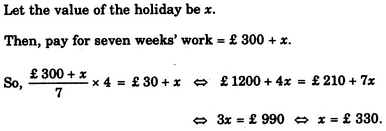Verbal Reasoning - Arithmetic Reasoning - Discussion

Discussion :: Arithmetic Reasoning - Section 1 (Q.No.34)

34.

Mr. Johnson was to earn £ 300 and a free holiday for seven weeks' work. He worked for only 4 weeks and earned £ 30 and a free holiday. What was the value of the holiday?

 [A]. £ 300 [B]. £ 330 [C]. £ 360 [D]. £ 420

Explanation:Paulwin Jerome said: (Feb 2, 2015) Let value of holiday = x. Pay for seven week = 300+x. Pay for one week = (300+x)/7. Pay for four week = 30+x. Pay for one week = (30+x)/4. Therefore (300+x)/7 = (30+x)/4. => 1200+4x = 210 + 7x => 990 = 3x => x = 330.

 Robin said: (Jan 20, 2017) How? Explain it detail & clear.

 Bruce said: (Oct 28, 2017) You are assuming that the values of two holidays are equal. Since the pay scale is not the same, so the value per day would not the same.

 Amol said: (Sep 7, 2020) Just calcutate the value. 7W + H = 300, 4W + H = 30. Solve the simultaneous equation. H 330.